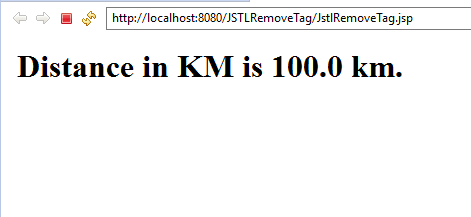# JSTL Core <c:if> Tag

The `<c:if>` tag is the most useful of tags of JSTL core tag library.JSTL `<c:if>` tag is a conditional tag used to evaluate conditional expression. When a body is supplied with c: if tag , the body is evaluated only when the expression is true.

The `<c:if>` tag evaluates an expression and displays its body content only if the expression evaluates to true.

The `<c:if>` tag is the most useful of tags of JSTL core tag library.JSTL `<c:if>` tag is a conditional tag used to evaluate conditional expression. When a body is supplied with c: if tag , the body is evaluated only when the expression is true.

The `<c:if>` tag evaluates an expression and displays its body content only if the expression evaluates to true.

## Syntax For c:if Tag

```<c:if attribute> body content </c:if>
```

## Attributes of <c: if> tag:

The `<c:if>` tag has following attributes:

• test :-Condition to evaluate.
• var :-Name of the variable to store the condition's result.
• scope :-Scope of the variable to store the condition's result.

## Example of c: if tag :

The bellow example will describe the real use of `<c:if>` tag.

Assume that you have a variable distance in your application. It is available in meter. You want to convert it to Kilometre. However you want to convert it only, if it is available and not null. You can use c:if tag in this case as given below.

```<%@ page language="java" contentType="text/html; charset=ISO-8859-1"
pageEncoding="ISO-8859-1"%>
<%@ taglib uri="http://java.sun.com/jsp/jstl/core" prefix="c"%>

<!DOCTYPE html PUBLIC "-//W3C//DTD HTML 4.01 Transitional//EN"
"http://www.w3.org/TR/html4/loose.dtd">
<html>
<meta http-equiv="Content-Type" content="text/html; charset=ISO-8859-1">
<title>JSTL If Tag Example</title>
<body>
<c:set var="distance" value="100000" />
<c:if test="\${distance != null}">
<h1>Distance in KM is is \${distance / 1000} km.</h1>
</c:if>
</body>
</html>
```

## Browser Output